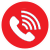# ISTQB – Equivalence Partitioning with Examples

## Equivalence Partitioning:

The word Equivalence means the condition of being equal or equivalent in value, worth, function, etc.    The synonyms for the word are equal, same, identical etc. Equivalence Partitioning is a black box technique to identify test cases systematically and is often the first technique to be applied when designing test cases.

Equivalence Partitioning is based on the idea that it in many cases the inputs/outputs to a program can be chunked into groups or classes which have the same behaviour.  Eg. Alphabets, Numbers, range of numbers etc

Let us consider a program that separates integers into positive or negative. And accepts any number between -5 and + 5.

The range of input integers can be split into  the following partitions :

• Negative Integers: Values between -5 and -1
• Zero (neither positive nor negative)
• Positive Integers: Values between  1 and  5
• Values >  5  (Invalid)
• Values <  -5 (Invalid)
 Valid Valid Valid Invalid Invalid -5         -1 0 1        5 > 5 < -5

Each of these is known as an ‘equivalence partition’ because every value inside the partition is exactly equivalent to any other value as far as the program is concerned. So -3 is exactly the same as -2, -1, -4, -5 and 1 is the same as 2,3,4,5. And testing with any one of these values is representative of the entire partition. Thus equivalence partitioning takes advantage of the properties of equivalence partitions to reduce the number of test cases.  Each equivalence partition covers a large set of other tests.

The first three partitions are called Valid partitions because they are within the range accepted by the program. The last two are called Invalid partitions because they are outside the range.  We can add more to the invalid partitions: decimal number partitions, alphabets etc.

The above are all examples of Input partitions because the partitions are based on the inputs to the program.

#### Output partitions:

Just as the inputs to a program can be partitioned, the outputs of a program could be partitioned.

Let us consider a bank account program that offers 0.5 percent interest for the first \$1000 savings, 1 percent for the next  \$1000 and 1.5 percent for the rest.

The outputs can be identified as: 0.5 % , 1 %, 1.5 %

 Invalid Valid – 0.5% Valid – 1% Valid 1.5% -0.01 \$, alphabets etc 0.00        1000.00 1000.01      2000.00 2000.01

Though the program mentions three partitions, we have identified four, 3 valid and one invalid condition. The Invalid partition will be used to test for proper error messages.

By identifying the partitions with cents  eg 1000 .01, the test cases add to the clarity of the program.

Summary:

Equivalence partitioning takes advantage of the properties of equivalence partitions or similarities/equivalences to reduce the number of test cases . This technique helps testers identify  the optimal number of test cases – ensuring test cases are not missed or randomly repeated. This is a more systematic method to writing test cases.

Steps to identify the test cases:

1. Identify the inputs/outputs from the specification eg. input fields in a form, inputs to command line programs, outputs-messages, calculations etc.

2. Identify the equivalence partitions or classes for the inputs/outputs identified

• For a boundary value – 1 valid equivalence partition (within the boundary) and one 1 invalid equivalence partition (outside the boundary).
• For a Boolean, 1 valid equivalence partition (true) and 1 invalid equivalence partition (false)
• For a range, 1 valid equivalence partition (within the range) and two invalid equivalence partitions(one outside each end of the range)
• If the input is a set of valid values, 1 valid equivalence partition (from within the set) and 1 invalid equivalence partition (outside the set)
• For a mandatory input, empty(invalid) and valid inputs.

3. Write test cases for the valid partitions followed by invalid partitions. There could be an overlap sometimes and new partitions identified as the test case design progresses.

This site uses Akismet to reduce spam. Learn how your comment data is processed.